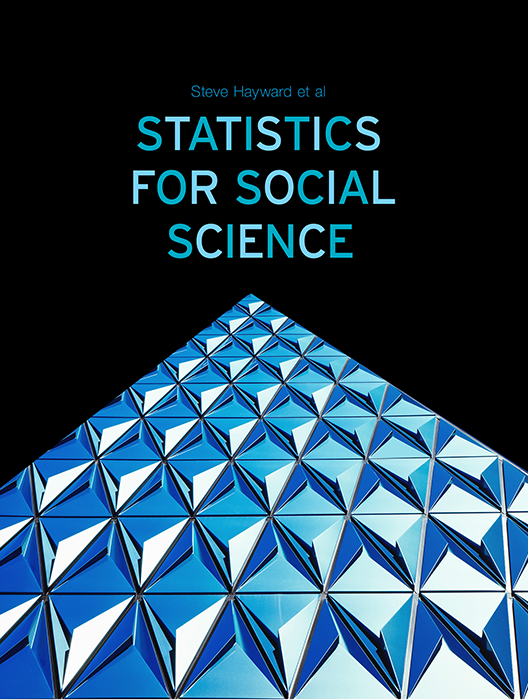# Statistics for Social Science

Lead Author(s): Stephen Hayward

Student Price: Contact us to learn more

Statistics for Social Science takes a fresh approach to the introductory class. With learning check questions, embedded videos and interactive simulations, students engage in active learning as they read. An emphasis on real-world and academic applications help ground the concepts presented. Designed for students taking an introductory statistics course in psychology, sociology or any other social science discipline.

This content has been used by 8,525 students

## What is a Top Hat Textbook?

Top Hat has reimagined the textbook – one that is designed to improve student readership through interactivity, is updated by a community of collaborating professors with the newest information, and accessed online from anywhere, at anytime.

• Top Hat Textbooks are built full of embedded videos, interactive timelines, charts, graphs, and video lessons from the authors themselves
• High-quality and affordable, at a significant fraction in cost vs traditional publisher textbooks

## Key features in this textbook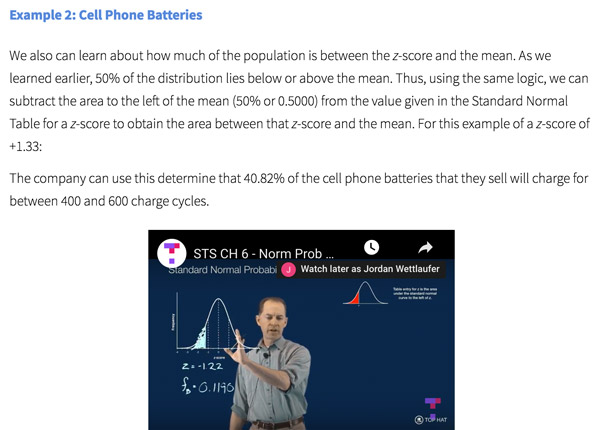Our Statistics for Social Science textbook allows students to manipulate data, visualize the effects discussed, and explore Lightboard videos that feature instructor explanations to reinforce concepts and calculations.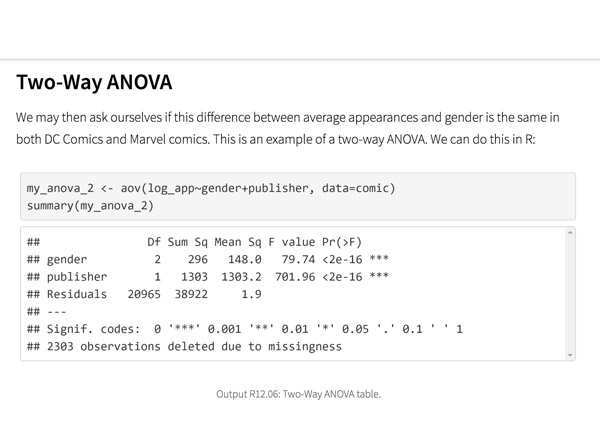Top Hat’s interactive offering includes a complementary module on using R software for data management, graphics, and conducting statistical analyses with examples and practice questions.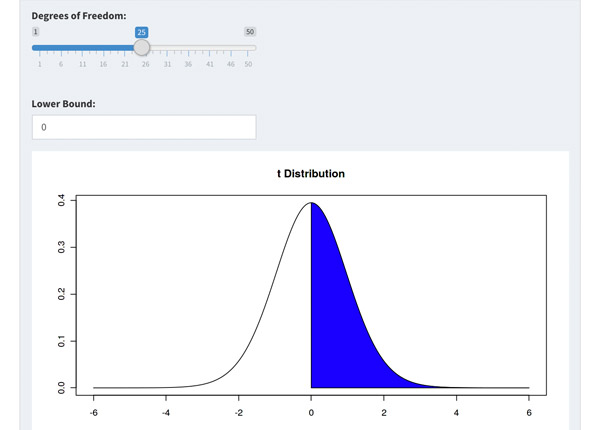Built-in assessment questions embedded throughout chapters so students can read a little, do a little, and test themselves to see what they know!

## Comparison of Social Sciences Textbooks

Consider adding Top Hat’s Statistics for Social Sciences textbook to your upcoming course. We’ve put together a textbook comparison to make it easy for you in your upcoming evaluation.

### Top Hat

Steve Hayward et al., Statistics for Social Sciences, Only one edition needed

### Pearson

Agresti, Statistical Methods for the Social Sciences, 5th Edition

### Cengage

Gravetter et al., Essentials of Statistics for The Behavioral Sciences, 9th Edition

### Sage

Gregory Privitera, Essentials Statistics for the Behavioral Sciences, 2nd Edition

### Pricing

Average price of textbook across most common format

#### Up to40-60%more affordable

Lifetime access on any device

#### $200.83 Hardcover print text only ####$239.95

Hardcover print text only

#### $92 Hardcover print text only ### Always up-to-date content, constantly revised by community of professors Content meets standard for Introduction to Anatomy & Physiology course, and is updated with the latest content ### In-Book Interactivity Includes embedded multi-media files and integrated software to enhance visual presentation of concepts directly in textbook Only available with supplementary resources at additional cost Only available with supplementary resources at additional cost Only available with supplementary resources at additional cost ### Customizable Ability to revise, adjust and adapt content to meet needs of course and instructor ### All-in-one Platform Access to additional questions, test banks, and slides available within one platform ## Pricing Average price of textbook across most common format ### Top Hat Steve Hayward et al., Statistics for Social Sciences, Only one edition needed #### Up to40-60%more affordable Lifetime access on any device ### Pearson Agresti, Statistical Methods for the Social Sciences, 5th Edition ####$200.83

Hardcover print text only

### Pearson

Gravetter et al., Essentials of Statistics for The Behavioral Sciences, 9th Edition

#### $239.95 Hardcover print text only ### Sage McConnell, Brue, Flynn, Principles of Microeconomics, 7th Edition ####$92

Hardcover print text only

## Always up-to-date content, constantly revised by community of professors

Constantly revised and updated by a community of professors with the latest content

### Top Hat

Steve Hayward et al., Statistics for Social Sciences, Only one edition needed

### Pearson

Agresti, Statistical Methods for the Social Sciences, 5th Edition

### Pearson

Gravetter et al., Essentials of Statistics for The Behavioral Sciences, 9th Edition

### Sage

Gregory Privitera, Essentials Statistics for the Behavioral Sciences, 2nd Edition

## In-book Interactivity

Includes embedded multi-media files and integrated software to enhance visual presentation of concepts directly in textbook

### Top Hat

Steve Hayward et al., Statistics for Social Sciences, Only one edition needed

### Pearson

Agresti, Statistical Methods for the Social Sciences, 5th Edition

### Pearson

Gravetter et al., Essentials of Statistics for The Behavioral Sciences, 9th Edition

### Sage

Gregory Privitera, Essentials Statistics for the Behavioral Sciences, 2nd Edition

## Customizable

Ability to revise, adjust and adapt content to meet needs of course and instructor

### Top Hat

Steve Hayward et al., Statistics for Social Sciences, Only one edition needed

### Pearson

Agresti, Statistical Methods for the Social Sciences, 5th Edition

### Pearson

Gravetter et al., Essentials of Statistics for The Behavioral Sciences, 9th Edition

### Sage

Gregory Privitera, Essentials Statistics for the Behavioral Sciences, 2nd Edition

## All-in-one Platform

Access to additional questions, test banks, and slides available within one platform

### Top Hat

Steve Hayward et al., Statistics for Social Sciences, Only one edition needed

### Pearson

Agresti, Statistical Methods for the Social Sciences, 5th Edition

### Pearson

Gravetter et al., Essentials of Statistics for The Behavioral Sciences, 9th Edition

### Sage

Gregory Privitera, Essentials Statistics for the Behavioral Sciences, 2nd Edition

## About this textbook

### Lead Authors

#### Steve HaywardRio Salado College

A lifelong learner, Steve focused on statistics and research methodology during his graduate training at the University of New Mexico. He later founded and served as CEO of Center for Performance Technology, providing instructional design and training development support to larger client organizations throughout the United States. Steve is presently lead faculty member for statistics at Rio Salado College in Tempe, Arizona.

#### Joseph F. Crivello, PhDUniversity of Connecticut

Joseph Crivello has taught Anatomy & Physiology for over 34 years, and is currently a Teaching Fellow and Premedical Advisor of the HMMI/Hemsley Summer Teaching Institute.

## Explore this textbook

Read the fully unlocked textbook below, and if you’re interested in learning more, get in touch to see how you can use this textbook in your course today.

# Two-Sample Hypothesis TestingTwo-sample​ tests are often used to test the effectiveness of pharmaceuticals. A sample of people will be given a particular dose of the drug, while another is given a different dose (or none at all). This enables pharmacists to discover the effectiveness of different doses of a drug. 

## Chapter Objectives

After completing this chapter, you will be able to:

• Determine the appropriate use of the z-test and t-test for two samples
• Distinguish between the z and t-distributions
• Distinguish between independent and dependent samples when comparing means of two populations
• Calculate and use z and t-test statistics in two-sample hypothesis testing
• Test the difference between two means
• Test the difference between two proportions

## Introduction

Two-sample hypothesis tests are used to make statistical decisions about relationships between two variables. Do students at public versus private high schools have higher or lower GPAs? Do lawyers have higher incomes than physicians? Are young adults more likely than older adults to support same-sex marriage? Are pet-owners less stressed than those who don’t own pets? Such questions go to the very heart of social research.

### What Comparisons Can Be Made?

There are a number of reasons for using statistical tests, but the purpose we will focus on in this chapter is to compare two parameters to see whether they are equal. More specifically, are they unequal enough to be statistically different? Can we reject a null hypothesis that the two statistics are equal and therefore represent separate and distinct populations?

What all these questions have in common is a comparison of statistics from two samples.

### What Statistical Test is Appropriate?

In this chapter, you will learn to compare means and proportions from two different samples using z-tests and t-tests.

## Comparisons of Means

Both z-tests and t-tests can be used for comparing means of interval and ratio level variables.

For example, we might be interested to see if men have higher mean incomes compared to women, or we might want to know if children’s mean number of words read per minute increases after an intensive reading lesson. In scenarios like these, we can use statistics to make decisions about whether the mean scores of two groups are reliably different.

Between-Groups Comparisons

These tests enable us to determine if a difference between two groups (e.g., students at public and private high schools, lawyers and physicians, younger and older adults) is large enough to be statistically significant. Is the difference large enough that we can reject the null hypothesis of no difference?

Within-Groups Comparisons

Other types of questions we can answer with two-sample hypothesis tests concern the effects of experimental interventions. Does relaxation therapy lower subjects’ blood pressure? Are injection drug users who receive harm reduction education less likely to share syringes? Does a short nap increase alertness? With these questions, we are comparing groups before and after the intervention. Is there a change? Is it in the hypothesized direction? Is it large enough to be statistically significant?

### Comparisons of Proportions

Z-tests can be used to compare proportions of nominal and ordinal level variables.

For example, we might want to know if Hawaii has a larger proportion of racial/ethnic minorities compared to Vermont, or whether the proportion of community college students in California who work full time is less than the corresponding proportion for Iowa. In cases like these, we can use statistics to make determinations about these parameters.

### What Determines the Approach?

Variations in the specific approach for these comparisons depend on:

• Size of the samples.
• Level of measurement of the variable of interest.
• Whether samples are independent or dependent
• Independent samples have no overlap. They are two mutually exclusive groups such as males and females or experimental and control groups.
• Whether the variances of the independent samples are equal (this does not apply to dependent samples).
• Dependent samples have some sort of overlap. For example, there may be two data points for the same person (perhaps pre- and post-test measures). There also might be pairs of data points for two people who are matched in the study, such as identical twins, married couples, or pairs of subjects with the same characteristics. We will address each of these scenarios in the following sections.
• Whether the standard deviation of the population (σ) is known or the sample standard deviation (s) is used to estimate it.

11.01

How many populations can we compare using z or t-tests?

11.02

What level(s) of measurement of variables can be analyzed using t-tests? (Select all that apply)

A

Nominal

B

Ordinal

C

Interval

D

Ratio

## Comparisons of Means

### Independent vs. Dependent Samples

Whether the two samples are dependent or independent is relevant when we are comparing two means. Simply put, independent samples are mutually exclusive, with no overlap or connection. Dependent samples have some overlap or connection to each other. This distinction affects how the means are compared and calculated, as we will see shortly.

• Between-groups comparisons are based on data from independent samples.
• Within-groups comparisons are based on data from dependent samples.

Example: Independent Samples

A social scientist wishes to see if men work longer hours per week, on average, compared to women. She uses a large national survey to test her hypothesis. The two samples (men and women) are mutually exclusive. There is no overlap. There is only one measure (hours worked) for each individual. She will compare the mean number of hours for men to the mean number of hours for women.

Example: Dependent Samples

A psychologist is interested to see if listening to classical music diminishes the perception of pain among adults. He uses 60 volunteers for his experiment. For half of the sample, he first pricks subjects’ big toes with a needle, then asks them to rate their level of pain on a scale of 0 (absolutely no pain) to 10 (unbearable pain). After a 60-minute break, he resumes the experiment by playing Mozart’s Serenade for Winds, 3rd Movement and giving the subjects time to relax and listen to the music. Then he pricks their toes again and asks them to rate their level of pain. He then tests to see if there has been a decrease in the perceived level of pain with the introduction of the music. He has two ratings of pain, sample 1 (no music) and sample 2 (music), but they are from the same group of 30 subjects. In this way the two samples are dependent. They are linked in terms of being two measures of pain for the same 30 individuals—two measures per person. To test his hypothesis, the psychologist will calculate a mean of differences for the two measures of pain.

### Determining the Test Statistic to Use

Which test statistic to use depends on two factors: 1) sample size, and 2) whether the population variance (and, therefore, the standard deviation) is known. Note that with an unknown standard deviation (σ), in order to use the standard normal distribution both groups must have an n ≥ 30; if either sample has n < 30, the t-distribution must be used instead. With a known Ϭ, the standard normal distribution can be used regardless of sample size.

Here is a decision table to use: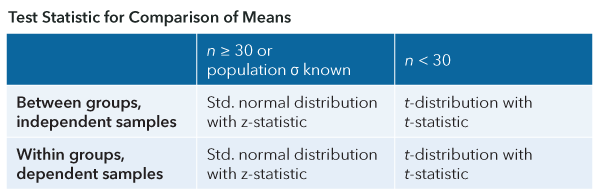Figure 11.1: Test statistic for comparison of means​​

## The Standard Error of the Mean

As we will see when we cover the formula for z and t, the standard deviation is used to calculate the standard error (SE) of the mean. This is a measure of variability of sample means around the population mean in a sampling distribution of means. In other words, a probability distribution is created for many values of means from many different samples. As the number of samples and/or the size of the samples increase, the standard error becomes smaller and smaller indicating little variation around the population mean. The smaller the standard error, the more precise the estimate of the population mean.

11.03

Which of the following examples is not a test with dependent samples?

A

Comparing mean life satisfaction scores for seniors (age 65+) and for middle-aged adults (ages 40-50).

B

Comparing pre- and post-test number of hours of sleep daily.

C

Comparing number of alcoholic drinks consumed in a typical day for pairs of twins.

D

Comparing the number of words read per minute by students before and after taking a speed-reading class.

11.04

A between-groups comparison of means involving samples of $n_1$ = 34 and $n_2$ = 42 with unknown σ would use the $\_\_\_\_$ - statistic as the test statistic.

## Large Sample Testing Using the z-Test

The distribution of possible z-scores is a hypothetical distribution in the shape of a bell or normal distribution. According to the central limit theorem, as the size of a sample (n) increases, the frequency distribution for any given variable (X) will begin to approximate a normal distribution. With the assumption of a normal distribution, z-scores can be used to test hypotheses. As illustrated in the following figure, the minimum n to be able to assume normality is 30.Figure 11.2: Changes in the shape of sampling distributions with increasing sample sizes​

Regardless of the original shape of a population distribution (panel A in the above figure) for any given variable (X), the resulting sampling distribution of the sample mean will become approximately normal in shape when the sample n is 30 (panel C) or greater. Note the change in shapes as n increases from 15 to 30. With a normal distribution, z is the appropriate test statistic since the distribution of z-scores is normal in shape

To be able to use z for testing the differences between two statistics, both of the two samples must be greater than or equal to 30 in size, i.e., n1 ≥ 30 and n2 ≥ 30, where n1 is the size of the first sample and n2 the size of the second sample.

Alternatively, z can be used if the variance of the population is known, but this is most often not the case when conducting hypothesis tests in the real world.

### z-Statistic

The probability associated with a difference between two means can be determined once the difference is standardized as a z-statistic. A calculated z-value with an associated probability in the “rejection region” in either of the two tails of the normal distribution would provide evidence that there is a difference between the two means. The null hypothesis that the means are equal can be rejected. The areas of rejection are shown in blue in the following figure.Figure 11.3: Rejection regions in the normal distribution​​

In the example above, the rejection region is located on both sides of the sampling distribution, that is, in the two tails of the distribution. Tests can also focus on only one of the two tails.

11.05

Click on the correct requirement to use z with two sample tests.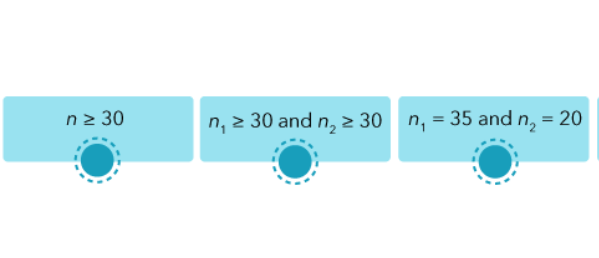### Critical Values of z

In order to test hypotheses using z, we look to the table of z-scores to identify the critical values with which we will decide whether or not we can reject the null hypothesis. A summary of commonly used critical values is presented here.Figure 11.4: Critical z-values for hypothesis tests​​

If the expected difference between means is negative (mean1 is less than mean2), then a statistically significant result for z would be negative and in the lower tail. If the expected difference is positive (mean1 is greater than mean2), then a statistically significant result for z would be positive and in the upper tail. If we expect there will be a difference between the two means but are not predicting that one will be larger than the other, a statistically significant result for z could be either positive or negative and could lie in either tail. This would constitute a two-tailed test. With a two-tailed test, we must split alpha (the rejection region of the distribution) between the two tails of the distribution. For example, a two-tailed test with an alpha of .05 has .025 in each tail (.05/2 = .025). The rejection regions are illustrated here.Figure 11.5: Upper-tail rejection region​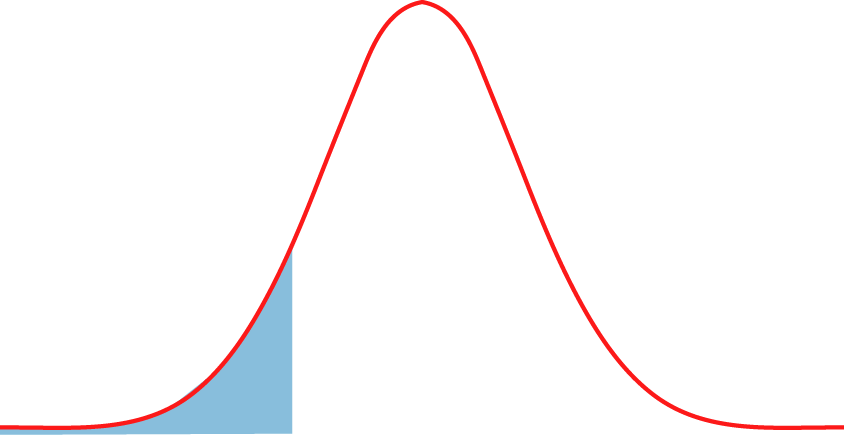Figure 11.6: Lower-tail rejection region​Figure 11.7: Two-tailed rejection region​

11.06

For a two-tailed test with an alpha of .05, click on the critical value(s).

A

1.645

B

1.96

C

-1.645

D

-1.96

E

2.32

## Small Sample Testing Using t-Test

The z-statistic is only appropriate if both samples have a sample size of 30 or greater, (or when the population variance is known). If one or both samples have an n that is less than 30 a t-test can be used instead.

The t-distribution is appropriate for smaller samples—those with less than 30 elements. As you learned, the t-distribution is a family of distributions whose shapes depend on their degrees of freedom (df). As a quick review, degrees of freedom refer to the number of values in a calculated statistic that are free to vary (do not have a fixed value).

Example: Degrees of Freedom

If you are given the first three numbers in a distribution of four numbers as 2, 4, and 5, and then must identify the fourth number so that all four numbers will add to 20, the fourth number must be 9. The first three numbers can vary, but once they are determined, the last number is no longer free to vary. So we say that this distribution has n – 1 degrees of freedom, or df = 3. The particular formula for degrees of freedom with t depends on the type of test being conducted—for example, one or two samples.

### Shape of the t-Distributions

The family of distributions looks like this:Figure 11.8: Family of shapes for the t-distribution

Notice that the shapes of the t-distributions with small sample sizes (n=1; n=2; n=4) are relatively flat, with an apex that’s lower than that of a normal distribution and tails that are higher or thicker. However, when n = 30, the shape of the t-distribution becomes very close to normal. For this reason, when n is 30 or greater, we can use z because the shape of the population distribution is approximately normal. When n is less than 30, the shape of the distribution may not be normal, so we must use t. Since we do not typically know the shape of the population distribution, this criterion of sample size for z and t provides the best estimates of the shape.

11.07

Click on the sample size(s) for which we would use t with two sample tests. (Select all that apply)

A

$n$ ≥ 30

B

$n_1$≥30 and $n_2$≥30

C

$n_1$=30 and $n_2$=20

11.08

The z-statistic is appropriate for hypothesis testing with which of the following samples (assuming that the population variance is unknown)?

A

$n_1$ > 30 and $n_2$ < 30

B

$n_1$ ​> 30 and $n_2$> 30

C

$n_1$< 30 and $n_2$ < 30

D

$n_1$ < 30 and $n_2$ > 30

### Critical Values of the t-Statistic

The table of critical t-values is more complicated than that of z-scores because the critical values of t depend on their degrees of freedom. Most tables of t found in statistics books arrange the critical values in a table with degrees of freedom indicated in the rows and alphas in the columns. An abridged version of a t-table is shown here.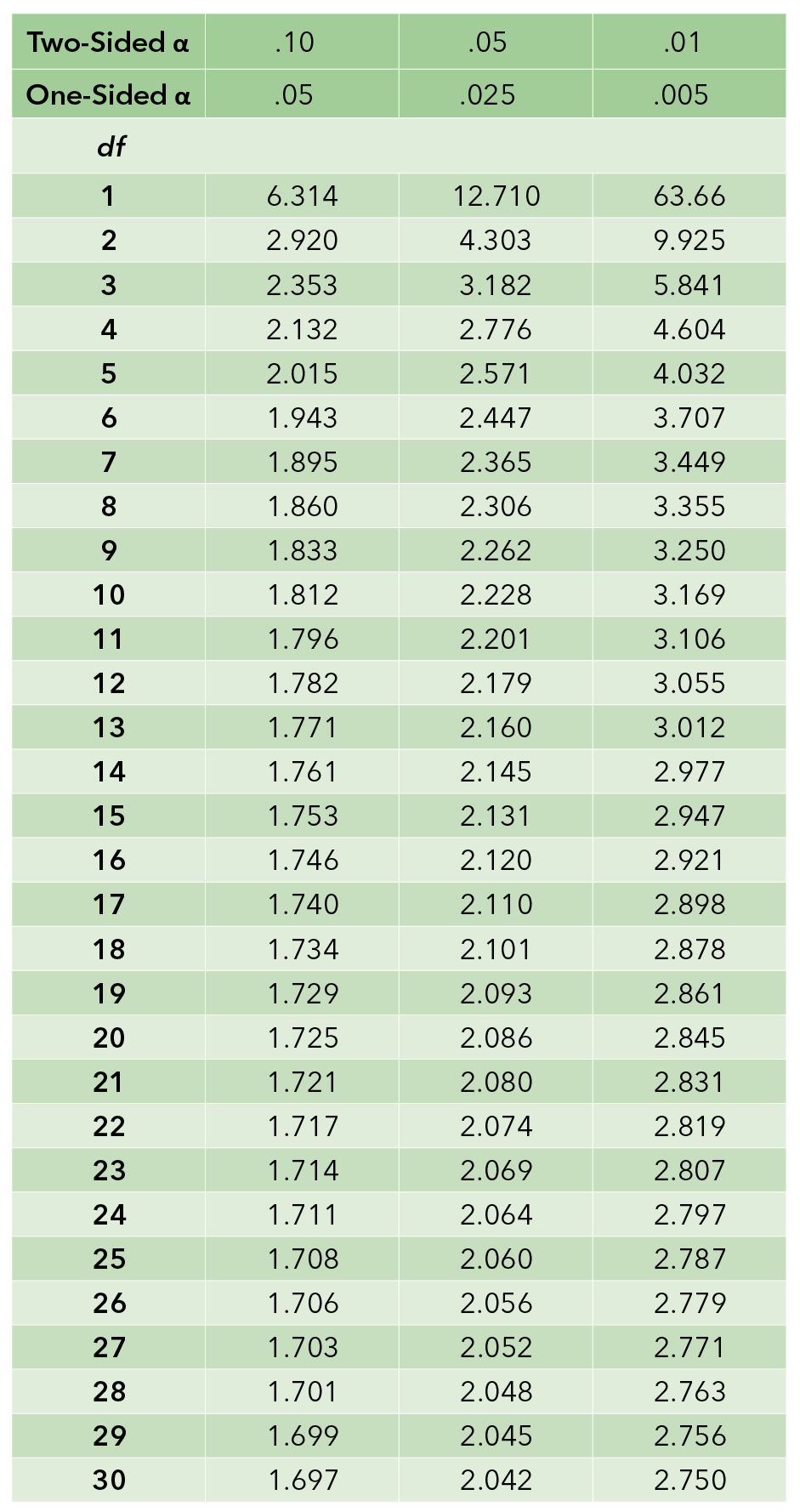Figure 11.9:​ Critical values of t​.

11.09

What is the critical t-value for a two-tailed test with alpha .05 and 18 degrees of freedom?

### Review of Hypothesis Testing

Before going through an example, here is a review of the steps of a hypothesis test.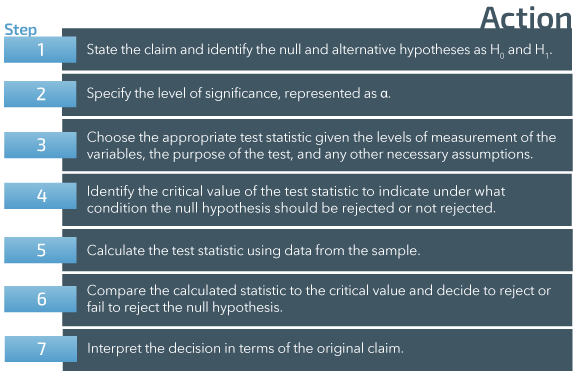Figure 11.10: Steps of a hypothesis test​

## Testing a Hypothesis about Two Means–Independent Samples

Recall that two independent samples are mutually exclusive. There is no overlap or connection between them. For example, we might compare mean scores for males vs. females, for seniors vs. middle-aged adults, or for urban vs. rural residents. In each case, we will compare two separate means, each obtained from a separate and non-overlapping group. More specifically, is there a statistically significant difference between the two different means? We can use either z or t for this test, depending on sample size and whether the population σ is known.

As we’ve seen, the central limit theorem provides evidence that as the sample size increases, the shape of the distribution for statistic X becomes more normal. If n is 30 or larger, we can assume normality and use z. If n is less than 30, we will use t. Because we are using two independent samples, both samples must meet the sample size criterion for the test that is to be used. If one or both samples are smaller than 30, then t is the statistic of choice. The formula is essentially the same for z and t. The difference lies in the probability distributions for the two statistics and the fact that the t-statistic requires the extra step of calculating the degrees of freedom. The basic formulas are:

According to the null hypothesis, the expected difference between population means is zero. This means the formulas can be simplified to:

### Figuring the Standard Error – Approximately Equal Variances

As a general rule of thumb, if one variance is no more than twice the other, they are considered approximately equal, and a pooled estimate of the standard error can be used. Under the assumption of equal population variances, the pooled sample variance provides a higher precision estimate of variance than the individual sample variances. This higher precision can lead to increased statistical power when used in statistical tests that compare the populations, such as the t-test.

To calculate the standard error (SE), start by figuring the pooled estimate of the standard deviation, represented as sp or spooled. It is a weighted average so that if one sample is much larger than the other, it will count for more. Note that s21 and s22 are the sample variances (not standard deviations) and n1 and n2 are the sizes of the two samples (1 and 2). The square root is taken to complete the calculation as the estimate of the pooled standard deviation.

The pooled estimate of the standard deviation is then used to calculate the standard error.

### Figuring the Standard Error – Unequal Variances

If one variance is more than twice the other, then the unpooled standard error is used. The rationale is that using a pooled estimate when the variances are "too different" has been shown to lead to unreliable results with the t-test (i.e., low p-values). "Too different" in this case is (somewhat arbitrarily) defined as the condition where one variance is more than twice the other.

### Degrees of Freedom

Recall that degrees of freedom indicate the number of measurements in a set that are free to vary. They are used in connection with t-statistics because the df, in turn, identify the particular t-distribution to be used in making a comparison. Just as with the standard error, the degrees of freedom can be either pooled or unpooled. Use the same criteria as above for determining which method to use.

Figuring Degrees of Freedom – Approximately Equal Variances

With two independent samples with approximately equal variances (one is no more than twice the other) the df are determined by summing the sample sizes and then subtracting the number of samples:

dfpooled = (n1 + n2) - 2

Figuring Degrees of Freedom – Unequal Variances

As with the standard error, if one variance is more than twice the other, then the unpooled df is used. It is rather complicated to calculate by hand and is best done using technology or an online calculator like the one here

In a pinch, some sources suggest using a conservative estimate such as this:

dfunpooled = the smaller of (n1 – 1) or (n2 – 1).

Using the smaller df leads to a more stringent test, as the p-value increases as the df decrease.

For a more exact computation, the complete formula is given below:

Example: Testing a Hypothesis about Two Means Using t

We’ll go through an example using t, which includes calculating the degrees of freedom. Other than the df calculation, an example using z would follow the same steps.

The social scientist interested in the hours worked by men and by women decides to conduct an analysis of employees in her department at Research Circle Institute. Her department employs 50 scientists (20 women and 30 men). Based on her general observations, she predicts that women will work longer hours per week compared to men. She surveys all 50 scientists concerning their typical number of hours worked per week and calculates the mean number of hours for the men and for the women. The means and standard deviations are:

• F = 50.9; M = 48.2
• sF = 1.1; sM = 0.9

Note that the means are distinguished by subscripts of female for females and male for males. Often times, the means are distinguished by numbers 1 and 2. A point to remember is that the alternative hypothesis determines the order of subtraction. For example, if the social scientist predicts that the mean for women will be greater than that for men, the mean for men would be subtracted from the mean for women, and the alternative hypothesis would be written as: H1: µfemale > µmale. She would expect a positive t-value and, consequently, would perform an upper-tailed, or right-tailed, test.

### Degrees of Freedom

With two independent samples, the sample sizes are summed, and the number of samples is subtracted from the sum:

The social scientist should determine her research hypothesis before she examines the actual mean values. In this example, if the mean for men is greater than that for women, obviously her hypothesis could not be supported. However, she does observe that the mean for women is greater than the mean for men, so she continues with the test. Women work longer hours than men, but is the difference large enough to be statistically significant? Can the null hypothesis of no difference be rejected?

Step 1: State the claim and identify the null and alternative hypothesis as H0 and H1.

Women work longer hours per week compared to men. Note that the symbols in the hypothesis statements are complementary.

Step 2: Specify the level of significance, represented as α.

She chooses a conservative level of significance with an alpha of .005.

Step 3: Choose the appropriate test statistic.

The correct test statistic is t because one of the independent samples is smaller than n = 30.

Step 4: Identify the critical value of the test statistic to indicate under what condition the null hypothesis should be rejected or not rejected.

The degrees of freedom for this test is (20 + 30) - 2 = 48. The critical value of t with an upper-tailed test, alpha of .005, and 48 degrees of freedom is 2.750 (see the t-table presented above). Note that the table only goes up to 30 degrees of freedom; this is the value chosen for 48 degrees of freedom. This is acceptable because the values of t increase in very small increments beyond 30 degrees of freedom. The null hypothesis will be rejected if t ≥ 2.750

Step 5: Calculate the test statistic.

First, she calculated the pooled standard deviation.

Second, she calculated the standard error:

Third, she used the differences between the means and the SE to calculate t:

Step 6: Compare the calculated statistic to the critical value and decide to reject or fail to reject the null hypothesis.

H0 is rejected because 10.00 > 2.75

Step 7: Interpret the decision in terms of the original claim.

She has support for her research hypothesis that women in her department work longer hours than do men. The difference is significant at the .005 level.

## P-Value Approach

An alternative way to approach this (and an increasingly common way in current research practice) is to calculate the P-value of t and compare that to the probability limit set by alpha. This provides more information than simply reporting a result as, for example:

t (48) = 10.00, p < .005

Using technology, the P-value associated with a t-statistic of 10.00 and df = 48 is P < .00001. Since the P-value is less than the alpha limit of .005, the null is rejected. Note that in this case, the P-value is extremely small because of the large df involved.

You can adjust the values in the demonstration below to see how the rejection regions and P-values change as a function of alpha and the value of the test statistic.This Content is Locked
Only a limited preview of this text is available. You'll need to sign up to Top Hat, and be a verified professor to have full access to view and teach with the content.

## Effect Size

The effect size is another useful result to include in a research report. It provides an easy-to-grasp measure of the actual size of the effect that was shown in a hypothesis test. A test statistic called Cohen’s d, arguably the most familiar approach to determining effect size, uses an estimate of the pooled standard deviation in the denominator of the formula, but it differs somewhat from the pooled estimate used in calculating the test statistic.

The formula for the pooled standard deviation is:

Combined, the two formulas can be shown as:

The value for d tells us the mean difference between the two groups is equivalent to 2.69 standard deviations, a very substantial difference given the range of possible hours of work per week. This would be reported as an extremely large effect size and supports the conclusion that there is a reliable difference in work hours.

11.10

Select the correct formula for the degrees of freedom for a t-test of the differences between two means with independent samples and approximately equal variances.

A

$n$-1

B

$k$-1

C

(r-1)(c-1)

D

($n_1$+$n_2$)-2

11.11

$S_{pooled}$ can be calculated using the sample size and standard deviations from the samples.

A

True

B

False

11.12

Given the same t-statistic value and the same variances, a hypothesis test based on larger samples would likely show a smaller effect size.

A

True

B

False

Example: Calculating t to Compare Two Means from Samples with Unequal Variances

A social epidemiologist wants to test a brief intervention to help people stop smoking cigarettes. He assigns 20 smoking volunteers to one of two groups—experimental and control. The experimental group (n1=10) are given an intervention about empowerment, and the control group watches movies on an unrelated topic (n2=10). After the intervention and movie-watching, he asks the subjects to record how many cigarettes they smoked in one day. He hypothesizes that the experimental group will smoke fewer cigarettes on average compared to the control group. The results are:

• Experimental group: x̄1 =16.1;   s1=3.2
• Control group: x̄2=18.4;   s2=1.1

He then tests his hypothesis by working through the following steps.

Step 1: State the claim and identify the null and alternative hypothesis as H0 and H1.

The experimental group will smoke fewer cigarettes in one day compared to the control group.

Step 2: Specify the level of significance, represented as α.

He chooses an alpha of .05.

Step 3: Choose the appropriate test statistic.

Since both samples have n less than 30, t is the correct test statistic. He tests to see if he can pool the variances:

Since variance 1 is more than 2 times the value of variance 2, he does not pool the variances.

Step 4: Identify the critical value of the test statistic to indicate under what condition the null hypothesis should be rejected or not rejected.

Since the variances are unequal, the researcher uses a technology tool to calculate the df as 11.09.

The critical value of t for a lower-tailed test with alpha of .05 and 11 degrees of freedom is -1.796.

H0 will be rejected if t ≤ -1.796

Step 5: Calculate the test statistic.

Step 6: Compare the calculated statistic to the critical value and decide to reject or fail to reject the null hypothesis.

The null hypothesis is rejected because -2.15 < -1.796.

Step 7: Interpret the decision in terms of the original claim.

The epidemiologist has evidence at alpha .05 that the experimental group smoked, on average, fewer cigarette per day compared to the control group

### P-Value Approach

An alternative way to approach this (and an increasingly common way in current research practice) is to calculate the p-value and compare that to the limit set by alpha. This provides more information than simply reporting a result as, for example:

Using technology, the p-value associated with a t-statistic of -2.15 and df = 11 is p = .027323. Since the p-value is less than the alpha limit of .05, the null is rejected.

### Effect Size

Recall that the effect is another useful result to include in a research report, providing an easy to grasp measure of the actual size of the effect that was shown in a hypothesis test. Cohen’s d, arguably the most familiar approach to determining effect size, uses an estimate of the pooled standard deviation in the denominator of the formula, but it differs somewhat from the pooled estimate used in calculating the test statistic.

In the epidemiological example above, the effect size could be calculated as:

The value for d tells us the mean difference between the two groups is equivalent to -0.96 standard deviations. This would be reported as a large effect size and supports the conclusion that there is a reliable difference in cigarettes smoked per day.

## Confidence Interval for the Difference between Two Means – Independent Samples

Another way to test for the equality of two means with independent samples is to calculate a confidence interval around the difference. It should be noted that the confidence interval approach assumes a two-tailed test. The exact formulas for the intervals vary depending on whether or not the variances are equal.

### Equal Variances

We have already seen how to calculate the components for this type of confidence interval. The formulas are:

Where the point estimate is the differences between the two means, t or z reflects the level of confidence, andf sp is the pooled standard deviation, which is multiplied by the square root of the summed fractions to calculate the standard error of the differences

11.13

What is the point estimate in the confidence interval for the difference between independent means?

A

$s_p$

B

$\frac{1}{n_1} + \frac{1}{n_2}$

C

$(x̄_1-x̄_2 )$

D

None of these

Example: Equal Variances

Let’s return to the example of the social scientist testing the difference in the mean number of hours worked per week between the women and men in her department. The data are:

Because we are calculating a confidence interval allowing values to be smaller and larger than the point estimate, we use the two-tailed t-value with an alpha of .01 (.005 x 2): 2.750.

Since the confidence interval does not include 0 (which we would see if the two means are equal), we can be 99% confident that the means are not equal. A comparison of the values shows the women worked longer hours on average than men in the scientist’s department.

### Unequal Variances

The confidence interval for two means from independent samples with unequal variances is slightly different than the one with equal variances because we can’t pool the standard deviations. We’ve already covered the components of the formula. Depending on whether z or t is being used, the formula for the confidence interval with two unequal variances is:

Notice that the formula does not include a pooled standard deviation value. Instead, the standard error is simply the square root of the sum of ratios of variance to sample size. So the only difference between the confidence intervals for equal and unequal variances is the standard error—including or not including a pooled standard deviation.

Adjust the sliders to see the effect of changing the mean and variance of the DV.This Content is Locked
Only a limited preview of this text is available. You'll need to sign up to Top Hat, and be a verified professor to have full access to view and teach with the content.

Example: Unequal Variances

Now return to the study testing a smoking cessation intervention. Because this study requires the t-test, the degrees of freedom for t must be calculated using the elaborate formula presented above. We won’t repeat that here. We’ll take advantage of already knowing the degrees of freedom and the relevant value of t. The data are:

Experimental group: x̄1=16.1; s1=3.2; n1=10

Control group: x̄2=18.4; s2=1.1; n2=10

t (α = .10; 11 df) = 1.796

Because the confidence interval does not include 0, we are 90% confident that the means are not equal. The experimental group smoked fewer cigarettes, on average, in a day than did the control group.

11.14

Match the standard error with the type of test.

Premise
Response
1

Comparing means with unequal variances

A

$\sqrt{\frac{s^2_1}{n_1}+\frac{s^2_2}{n_2}}$

2

Comparing means with equal variances

B

$s_p\sqrt{\frac{1}{n_1}+\frac{1}{n_2}}$

C

$\frac{s}{\sqrt{n}}$

### Comparing Confidence Intervals for Two Means

If we already know the confidence intervals around the two independent means, we can compare the intervals to see if there is overlap to determine whether or not they are equal. Do the intervals share any values of the means? With this approach, we don’t need to calculate the difference between means or worry whether or not the variances are equal. The confidence intervals are calculated separately for the two samples.

The confidence interval for means is:

How do we use confidence intervals to test the difference between means? We calculate both intervals (around each of the means) and compare them looking for overlap where both intervals contain the same value or values of the estimated mean. If both intervals contain the same value of the means, then we can’t be confident that the means are not equal. The means could be equal given the margins of error.

Say these brackets represent two confidence intervals for two means:Figure 11.11: Mean values of X1 and X2​

The shaded areas indicate overlap of the potential value of the means in the intervals. The upper end of the interval on top shares the same values as the lower end of the interval below it. With these two confidence intervals, we can’t be sure at the chosen level of confidence that the two means are not equal. If the actual values of the means fall at the same point in the shaded areas (as indicated by the line), the means are equal.

11.15

When comparing two different confidence intervals around two means, we conclude that the means are not equal if the intervals overlap or don’t overlap?

A

overlap

B

don't overlap

11.16

Click on the standard error in a confidence interval around one mean.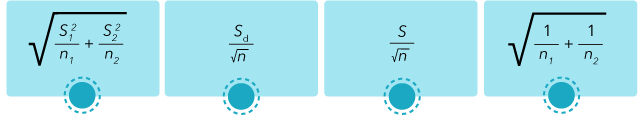Examples: Comparing Confidence Intervals for Two Means

Let’s repeat an earlier example of hypothesis testing the differences between means with the confidence interval approach to see if we come to the same conclusion about the means equal or not. We’ll return to the social scientist’s comparison of hours worked per week by men and women. Recall that her hypothesis test showed that the women in her department worked longer hours, on average, compared to the men. Let’s calculate and compare the two confidence intervals. The data are:

Recall that we used t because the sample of women had fewer than 30. The degrees of freedom is (20 + 30) – 2 = 48. The social scientist chose an alpha of .005 for a one-tailed test. Because we are calculating a confidence interval allowing values to be smaller and larger than the point estimate, we use the two-tailed t-value with an alpha of .01 (.005 x 2): 2.750.

The confidence interval for women’s mean number of hours worked per week is:

The confidence interval for men’s mean number of hours worked per week is:

Comparing the two confidence intervals, there is no overlap. They are mutually exclusive. The lowest value for the women’s interval is 52.21, and the highest value for the men’s interval is 48.64. Clearly, the intervals don’t share any of the same values. So these results match those of the hypothesis test presented earlier. We are 99% confident that the means are not equal. Examining the two values, we conclude that women work longer hours than men.

## Testing a Hypothesis about Two Means – Dependent Samples

In psychological research, dependent samples are often used to compare scores before and after treatments, or when the researcher needs to pair subjects to either control for differences or to be able to match them based on a relevant characteristic (i.e. twins, married couples, and so on). For example, participants may be tested to obtain scores on a measure, then exposed to a treatment and later retested to see if their scores have changed. If so, the researcher may be able to infer an effect due to the treatment that was delivered. Participants might also be paired for the analysis.

### Step-by-Step Overview

Step 1: The null hypothesis for this test is that the mean of the differences will be zero (0). In other words, there is no difference in scores or values between the two dependent samples. In the hypotheses, as usual, the mean is expressed as a population parameter (µd).

Step 2: The alpha level is chosen.

Step 3: Choose the correct statistic for comparing means for two dependent samples. Even though we consider this test to have two samples, in reality the sample size is defined in terms of the number of pairs being compared. So n = number of pairs. Typically, this type of study includes a limited number of pairs. The sample size is usually less than 30, so t is typically the appropriate test statistic. However, if the study has 30 or more pairs (n ≥ 30), z should be used as the test statistic.

Step 4: Choose the critical value of t. The degrees of freedom for comparing two means that are paired, or dependent, is (n - 1). Stated another way, it is the number of pairs minus 1.

Step 5: Calculate the test statistic. The relevant formula for t is:

Step 6: Compare the calculated statistic to the critical value and decide to reject or fail to reject the null hypothesis.

Step 7: Interpret the decision in terms of the original claim.

### Terms and Symbols

Below is a table with a summary of relevant terms and symbols: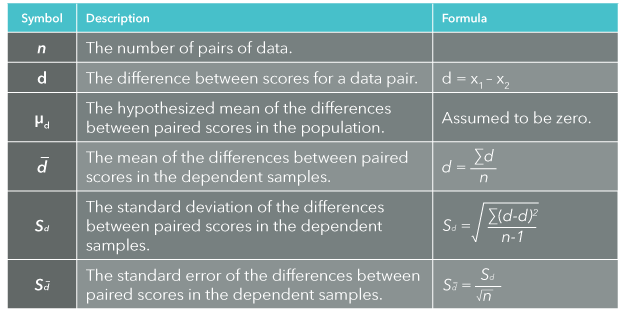Figure 11.12: Terms and symbols for calculating statistics comparing dependent samples​

### Calculation Procedure

The basic steps for conducting a t-test for the means of dependent samples are summarized in the following table.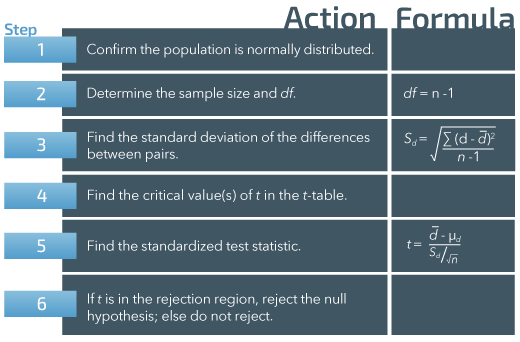Figure 11.13: Action and formula for testing hypotheses for dependent samples​​
11.17

Match the research hypothesis with the type of test.

Premise
Response
1

Two-tailed test

A

$H_1: µ_d ≠ 0$

2

Upper-tail test

B

$H_1: µ_d < 0$

3

Lower-tail test

C

$H_1: µ_d > 0$

D

$H_1: µ_d = 0$

11.18

In the dependent samples hypothesis test, the null hypothesis always states that µ$_d$ is equal to what value?

### The Mean of Differences

With two dependent samples, our statistic of interest is the mean of differences. What is the average difference for two measurements per person or within pairs for the same variable? A typical scenario for this type of test is a pre- and post-test measure—before and after measures of the same variable for each individual in the sample. How did the values change from pre- to post-test, on average for the whole sample? The formula for calculating the mean of the differences is:

Where d̅ is the mean of the differences for two measurements of X and the sum of differences is the mathematical difference (increased, decreased, stayed the same) in the two measures of X for each person. Let’s work through an example to help illustrate this calculation.

Example: Calculating the Mean of Differences

A cognitive psychologist believes that IQ test results are social constructs (defined by individuals’ experiences in their social world) rather than a true measure of intelligence, per se. He compared the IQs of adult identical twins and hypothesized that their IQ scores would be significantly different because no two twins can have the exact same social experiences. He does not expect one particular twin to have a higher or lower score.

This is an example of two dependent samples since the subjects are paired and comparisons are made within the pairs. His results of IQ measures are shown in the table below.

The first step in the calculation is to subtract the IQs within pairs as a way to measure their differences. The differences are then added together, and the sum of the differences is divided by the number of pairs to calculate d̅ (d-bar), the average of the pair-wise differences. Typically, the secondly occurring measurement is subtracted from the first, especially if the intention is to measure change that occurs over time.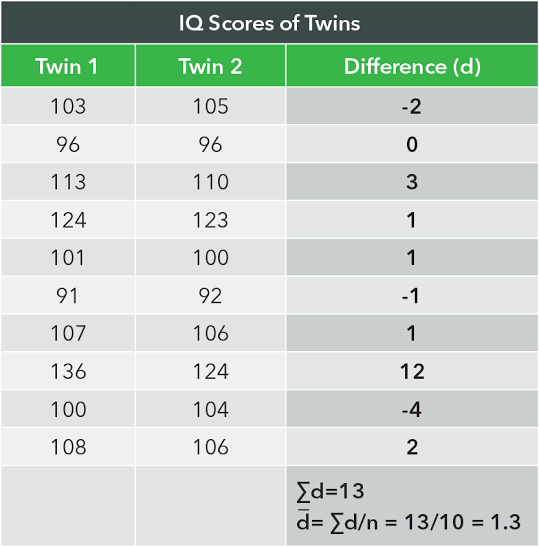Figure 11.14: Data for the IQ study example

Example: Calculating the Sum of Squared Differences from the Mean

Continuing with the twin study of IQs, we have calculated the mean of differences to be 1.3. Next, expand the table of paired scores and their differences. Note how similar this is to the process for calculating a variance.

Example: Completing the Hypothesis Test

With this information, the hypothesis test can be completed by following the hypothesis testing steps:

Step 1: State the claim and identify the null and alternative hypotheses as H0 and H1.

Pairs of identical twins will have different IQ scores.

Since this is a two-tailed test, the mean of the differences and the calculated t can be either positive or negative. The value can be in either tail of the t distribution.

Step 2: Specify the level of significance, represented as α.

The psychologist chooses an alpha of .05.

Step 3: Choose the appropriate test statistic

The appropriate test statistic is t because the number of pairs n = 10.

Step 4: Identify the critical value of the test statistic to indicate under what condition the null hypothesis should be rejected or not rejected.

The critical t has df = 9 (10 - 1 = 9). With a level of significance of .05, 9 degrees of freedom and a two-tailed test, the table of t values presented earlier indicates that the critical value is 2.262. The decision rule is:

Reject H0 if t ≤ -2.262 or if t ≥ 2.262.

Step 5: Calculate the test statistic.

Next, calculate sd

t can then be calculated as:

Step 6: Compare the calculated statistic to the critical value and decide to reject or fail to reject the null hypothesis.

t = 0.96 is not less than -2.262 or greater than 2.262. The null hypothesis cannot be rejected.

Step 7: Interpret the decision in terms of the original claim.

The psychologist cannot conclude that the pairs of twins in his study have significantly different IQs.

## Confidence Interval for the Difference between Two Means – Dependent Samples

Another way to test for the difference between two means with dependent samples is to calculate a confidence interval around the mean of the differences. Simply put, if the interval contains zero (0), then we can’t be confident that the there is a difference. The actual value of the mean of differences might be 0 if the interval includes 0. In other words, there is no difference, and the null hypothesis would not be rejected.

In this application, the point estimate is the mean of differences. The formula for this interval is:

Where d̅ is the mean of differences, sd is the standard deviation of the differences, and

is the standard error of the mean of differences. We use z if ≥ 30 and t if < 30. The margin of error for confidence intervals around the mean of differences includes two components:

• The value of z or t that reflects the level of confidence chosen for the interval.
• The standard error of the mean of differences includes the standard deviation of the population if known or of the sample if the population standard deviation is not known. Typically, the population standard deviation is not known, so s is used as an estimate. The standard deviation is then divided by the square root of n.

11.19

Arrange the formula for the confidence interval for the mean of differences in the proper order.

A

$\frac{s_d}{\sqrt{n}}$

B

$d̅$

C

$z$ or $t$

D

±

11.20

Click on the margin of error for the confidence interval around the mean of differences.11.21

Changing from a confidence level of 95% to 99% will do which of the following to the confidence interval?

A

Increase the confidence and increase the precision

B

Decrease the confidence and increase the precision

C

Increase the confidence and decrease the precision

D

Decrease the confidence and decrease the precision

Example: Confidence Interval for the Mean of Differences

Let’s return to the example twin study of IQ and see if we come to the same conclusion with the confidence interval approach as we did with the hypothesis test. The data are:

Since the interval does include 0 (which means no difference), we cannot be 95% confident that the mean of differences is not 0.

## Testing a Hypothesis about Two Proportions

Hypothesis testing can also be used to test the difference between two proportions. This involves comparisons between nominal or ordinal level variables, whereas testing the difference between means requires interval or ratio level variables. We can compare proportions of Democrats and Republicans who support gun control, of married and single adults who get at least 7 hours of sleep per night, or of men and women who own poodles. Unlike comparisons of means, proportions are always compared for two independent samples. For proportions there is no comparable measure to a mean of differences.

For this test, we use only z and only if at least 5 cases in each category of the variables of interest are within each of the two samples. The size can be determined as follows.

Min [n1 (p1), n1 (1-p1), n2 (p2), n2 (1-p2)] ≥ 5

Where n1 and n2 are the sample sizes of the two groups, p1 and p2 are the proportions of interest (say, smokers) and (1-p1) and (1-p2) is the proportion in the other category (say, non-smokers) of the variable. The proportion multiplied by the sample size is the count or frequency in the category of interest. Sample size multiplied by (1-p) is the frequency in the other category. Both categories in both samples must have frequencies of at least five (5).

For example, if

p1=.6, n1=10, p2=.4, n2=10,

then

(.6)10=6, (1-.6)10=4, (.4)10=4, (.6)10=6,

and we cannot conduct the test because the criterion of frequencies of at least 5 in all categories is not met.

The formula for comparing two proportions with z is:

In the numerator we calculate the difference between two proportions. The denominator is the standard error for the distribution of proportions. This is a pooled estimate because the formula contains the value for p. This is the proportion of interest for both samples combined or

Remember that this is the total p for both samples combined.

### Hypothesis Test for the Difference between Proportions

Step 1: Make the claim about the proportions. State the null and alternative hypotheses.

The null hypothesis is that the two proportions are equal. There are three possibilities for the alternative hypothesis: 1) proportion 1 is greater than proportion 2; 2) proportion 1 is less than proportion 2, or 3) the two proportions are not equal. The symbol for the population proportion is P, which is used in stating the hypotheses.

Step 2: Alpha is chosen.

Step 3: Choose the appropriate test statistic.

z is the test statistic of choice as long as the samples are independent and there are at least 5 cases in every category of the variable in each group.

Step 4: The critical value of z is chosen depending on the alpha level and whether the alternative hypothesis indicates an upper-tailed, lower-tailed, or two-tailed test.

Step 5: Calculate z.

Step 6: Compare the calculated z to the critical value of z to determine whether to reject or fail to reject the null hypothesis.

Step 7: Interpret the result relative to the original claim.

11.22

To test the difference between proportions, at least how many cases must each category of the variable for both samples have?

11.23

Which of the following is not included in the formula for z comparing two proportions?

A

Proportions for both samples

B

The overall $n$ (both samples combined)

C

The $n$ for both samples

D

The overall proportion (both samples combined)

11.24

Select the symbol for the population proportion.

A

B

μ

C

Ʃ

D

π

E

α

Example: Comparing Two Proportions

A historian is interested to see if the civil rights movement in the 1960s had an immediate effect on people’s positive or negative opinions of interracial marriage. She located a national opinion survey that’s been ongoing since 1952. The survey is conducted every year and includes independent or new samples every year. She compared the opinions of 1000 respondents in the 1952 survey to 1000 in the 1968 survey (the year after the Supreme Court ruled that it is unconstitutional to prohibit interracial marriage). In 1952, 260 out of 1000 respondents had a positive opinion about interracial marriage, and 740 had a negative opinion. In 1968, the numbers were 382 positive and 618 negative. This means that the proportion positive in 1952 was p1=.260, and the proportion positive in 1968 was p2=.382. The overall proportion for both samples combined is p = (260+382)/(1000+1000) = .321. She begins the hypothesis test…

Step 1: State the claim and identify the null and alternative hypothesis as H0 and H1.

Survey respondents in 1952 will have less favorable opinions about interracial marriage compared to those in 1968.

Step 2: Specify the level of significance, represented as α.

She chooses an alpha of .01.

Step 3: Choose the appropriate test statistic.

z is the correct test statistic because the samples are independent and both have a sample size of 1000. The opinion survey used a random selection method for the sample.

Step 4: Identify the critical value of the test statistic to indicate under what condition the null hypothesis should be rejected or not rejected.

With a lower-tail test and an alpha of .01, the critical value of z is -2.326. The decision rule is H0 will be rejected if ≤ -2.326.

Step 5: Calculate the test statistic.

Step 6: Compare the calculated statistic to the critical value and decide to reject or fail to reject the null hypothesis.

H0 is rejected because -6.100<-2.236.

Step 7: Interpret the decision in terms of the original claim.

There is evidence at alpha of .01 that the proportions are not equal. Comparing the sizes of the proportions, survey respondents in 1952 had less positive opinions about interracial marriage than those in 1968.

## Summary

In this chapter, we covered hypothesis tests for the differences between means and between proportions as well as confidence intervals for mean differences. At the beginning of the chapter, we noted that variations in the specific approach for these comparisons depend on the following:

• Size of the samples
• Whether samples are dependent on (there’s overlap between the two) or independent of (there is no overlap) each other.
• Whether the variances of independent samples are equal or not (does not apply to dependent samples).
• Level of measurement of the variable of interest.

These different approaches are summarized in the table below.Figure 11.16: Summary of goals and criteria for two-sample hypothesis tests​​​

## Case Study: Online vs. Traditional Classroom Environments

The following link is to an interesting study comparing the performance of students who took a statistics class online compared to those who took it in the traditional face-to-face classroom.

Read the entire short article, paying attention to the use of t-tests for independent and dependent (paired) samples. The author presented P-values for the t-tests but didn't present the actual t-values.

### Case Study Question 11.01

Case Study Question 11.01

Calculate the appropriate t-statistic for the means presented in table 1.

Click here to see the answer to Case Study Question 11.01.

### Case Study Question 11.02

Case Study Question 11.02

What additional information would you need to calculate t for table 2?

Click here to see the answer to Case Study Question 11.02.

### References

Schou, S. B. (2007). A Study of Student Attitudes and Performance in an Online Introductory Business Statistics Class. Retrieved from Electronic Journal for the Integration of Technology in Education.  Access the article at this link.

## Pre-Class Discussion Questions

### Class Discussion 11.01

Class Discussion 11.01

Create a research question for which it would be appropriate to use the test of mean difference—dependent samples. What are the null and research hypotheses?

Click here to see the answer to Class Discussion 11.01.

### Class Discussion 11.02

Class Discussion 11.02

Create a research question for which it would be appropriate to use the test of mean difference—independent samples. What are the null and research hypotheses?

Click here to see the answer to Class Discussion 11.02.

### Class Discussion 11.03

Class Discussion 11.03

What is the main difference in calculations for mean difference—independent samples when the variances are equal and the variances are unequal?

Click here to see the answer to Class Discussion 11.03.

### Class Discussion 11.04

Class Discussion 11.04

Create a research question for which it would be appropriate to use the test of proportion difference. What are the null and research hypotheses?

Click here to see the answer to Class Discussion 11.04.This Content is Locked
Only a limited preview of this text is available. You'll need to sign up to Top Hat, and be a verified professor to have full access to view and teach with the content.

## Answers to Case Study Questions

### Answer to Case Study Question 11.01

In Table 1, the variances are not equal (more than 2 times different), so the unpooled standard error should be calculated.

With about 14 degrees of freedom (15-1), the p-value is between 0.10 and 0.20.

Click here to return to Case Study Question 11.01.

### Answer to Case Study Question 11.02

For Table 2, individual scores for each participant are needed to calculate the mean of differences between pre- and post-test.

Click here to return to Case Study Question 11.02.

## Answers to Pre-Class Discussion Questions

### Answer to Class Discussion 11.01

The research question should focus on the comparison of dependent samples such as pre-and post-test measures, matched measures for paired samples, and cross-over trials. The dependent variable must be interval/ratio.

The null hypothesis would state a mean difference of 0. The research hypothesis can be one- or two-tailed.

Click here to return to Class Discussion 11.01.

### Answer to Class Discussion 11.02

The research question should focus on the comparison of means for two separate samples or mutually exclusive groups such as experimental and control, male and female, young and old. The dependent variable must be interval/ratio.

Click here to return to Class Discussion 11.02.

### Answer to Class Discussion 11.03

The main difference is the calculation of the standard error and degrees of freedom if it’s a t-test. When the variances are equal, the pooled standard error is calculated. When they are not equal, the unpooled measure is used. The degrees of freedom for equal variances is straightforward (sum both ns and subtract 2) but a more complicated formula or the smaller n-1 as an alternative is used for unequal variances.

Click here to return to Class Discussion 11.03.

### Answer to Class Discussion 11.04

The research question should focus on the comparison of two proportions from independent samples or mutually exclusive samples. The dependent variable must be nominal or ordinal.

The null hypothesis would state that the proportions are equal. The research hypothesis can be one- or two-tailed.

Click here to return to Class Discussion 11.04.

## Data Gathering Question

To the nearest tenth, what was your final GPA in high school?

_._ (fill in the number)

To the nearest tenth, what is your current GPA in college?

_._ (fill in the number)